• 发帖数1894
• 粉丝24

# [java/javascript教程] 通过代码示例学习 JavaScript 中的数据结构

### 登录网站，浏览更多精彩内容

x1 s8 e! T- b* j' [% k, T; P2 W9 i

const arr = [1, 2, 3]

// 每一个值都是彼此相关联的，因为每一个值都在数组中具备自己的索引序号
const indexOfTwo = arr.indexOf(2)" D+ [9 q1 t+ _% N6 k
console.log(arr[indexOfTwo-1]) // 1) x4 G" o- l1 H, ^3 t6 i  J! }\$ C
console.log(arr[indexOfTwo+1]) // 3

// 我们可以对数组进行很多操作，例如给数组添加一个新的值5 M\$ V* `- H\$ T# t. y
arr.push(4)
console.log(arr) // [1,2,3,4]
JavaScript 包含原始（内置） 和 非原始（非内置） 两种数据结构。原始数据结构是编程语言默认的、可以拿来就用（如数组和对象）的；而非原始数据结构不是默认的、如果需要使用的话，你必须先编写出来。不同的数据结构对应不同的操作场景。你或许可以使用内置数据结构处理大部分编程任务，但当遇到特殊任务的时候，非原始数据机构可以派上用场。让我们一起来看一看最流行的数据结构，它们是怎么运行的，在哪些场合适用，以及如何使用 JavaScript 编写这些数据结构。数组数组是存储在连续内存位置的项目合集。数组内的每一个元素都可以通过其索引（位置）访问。数组的索引通常从 0 开始，所以在一个包含 4 个元素的数组中，第三个元素的索引为 2。const arr = ['a', 'b', 'c', 'd']4 Y% }# Z\$ W& Y8 z# V% X
console.log(arr) // c9 [2 j% P# C. ~9 |& V; |

console.log(arr.length) // 4# f8 J7 i/ t5 z0 s4 C

JavaScript 数组可以存储任何数据类型的值，也就意味着可以存储数组。一个包含其他数组的数组被称为多维数组。const arr = [
[1,2,3],
[4,5,6],0 a. d* l5 b: K\$ E8 K- D1 `3 \
[7,8,9],
]
JavaScript 数组有许多内置的属性和方法，可以针对不同目的来使用，如从数组添加或者删除元素、给数组排序、过滤数组，以及我们知道的数组长度等，数组的完全属性和方法列表可以在这里找到。&#128521;在数组中每一个元素都对应一个索引，索引跟元素位于数组位置相关。如果我们在数组末尾添加一个新的元素，则这个元素的索引为之前数组最后一位索引加一。但当我们想要在数组的开头或者中间添加或者删除元素的话，添加或删除的这个元素之后的所有元素的索引都会变化。这样会增加计算成本，也是这种数据结构的缺点之一。当需要存储独立值以及在数据结构末尾添加和删除值的时候，数组十分有效。但当需要在结构中添加删除元素，其他数据结构会更有效。（我们会在后文提到）对象（哈希表）在 JavaScript 中，对象是键值对的集合。在其他编程语言中，这种数据结构也被称作映射、字典和哈希表。一个典型的 JS 对象如下：const obj = {
prop1: "I'm",
prop2: "an",
prop3: "object"
}4 y/ S* t) \0 o% p0 a' O9 y

prop1: "Hello!",
prop3: function() {console.log("I'm a property dude!")3 }( J7 R' {+ M: x
}}

console.log(obj["prop1"]) // "Hello!"
obj.prop3() // "I'm a property dude!"

obj["prop5"] = "The new prop on the block") Q; @1 E  p; t" a
obj.prop6 = () => console.log("yet another example")
% @( \# ]  j( @' \: x; ^7 W
console.log(obj.prop4) // 1256 K* e: W  J0 j& z7 y: a
console.log(obj["prop5"]) // "The new prop on the block"9 |+ _+ x# t, i\$ d9 N
obj.prop6() // "yet another example"! J0 F8 E! H2 R# w; v8 t

pizzaLovers: 1000,) U, }# H* r* h" T* k  w
pastaLovers: 750,
argentinianAsadoLovers: 12312312312313123, o4 H8 t- H: O3 @8 @; F% J0 |
}一叠纸只要确认元素遵循 LIFO 模式，那么栈结构就可以派上用场。下面是栈的使用场景：JavaScript 的调用栈在各种编程语言中管理函数调用许多程序提供的撤销/重做功能有不止一种实现栈的方法，但是最简单的或许是在数组中使用 push 和 pop 方法。如果你仅通过 pop 和 push 的方法来添加和删除元素，你就遵循了 LIFO 模式，用栈的方法操作了数组。另一个方法是列表，实现如下：// 为栈的每一个节点创建一个类1 `+ |, p' p9 _) q, s0 F; E' p
class Node {
// 每一个节点包含两个属性，其值以及一个指向下一个节点的指针) U) s" T8 z+ _4 b* ]% Y" e" Y
constructor(value){, w8 e3 ^/ s1 c( X6 K
this.value = value
this.next = null
}! v6 V) u! ^6 W
}
. F  ^# Z3 a5 S  @- X
// 为栈创建一个类- ^9 p, l, D  J6 Z\$ w
class Stack {* {4 f\$ v" c- I# o) S' T8 ~
// 栈有三个属性，第一个节点，最后一个节点，以及栈的大小
constructor(){
this.first = null: Q- l4 f) k9 z; W2 e* E
this.last = null
this.size = 0: [' U6 X5 y1 s, B9 n
}
// push 方法接受一个值，并将其添加到栈的“顶端”
push(val){
var newNode = new Node(val)
if(!this.first){
this.first = newNode8 I7 |2 }9 H6 p; @4 @% h) G
this.last = newNode
} else {
var temp = this.first
this.first = newNode
this.first.next = temp
}5 Q+ w/ B& q% B( B2 H4 S
return ++this.size, _0 R1 ~5 o  b. T1 _4 o
}
// pop 方法删除栈“顶端”的值，并返回这个值
pop(){
if(!this.first) return null
var temp = this.first
if(this.first === this.last){
this.last = null
}5 f  ]) E' c6 g6 x8 r\$ w
this.first = this.first.next
this.size--3 B\$ _2 I2 J( w8 }8 E5 [
return temp.value
}
}
; Y7 Y  y' L- S# h7 R5 T3 T
const stck = new Stack

stck.push("value1")
stck.push("value2")- y4 @3 ~0 l- T; i1 E4 ~2 `
stck.push("value3")

console.log(stck.first) /*
Node {
value: 'value3',6 e7 T! Z; f7 N: W; _
next: Node { value: 'value2', next: Node { value: 'value1', next: null } }
}
*/
console.log(stck.last) // Node { value: 'value1', next: null }
console.log(stck.size) // 3. F  Z' \. u\$ m( a\$ |

stck.push("value4"): l8 ?! b3 F  n5 {/ H
console.log(stck.pop()) // value4一队列的顾客队列的使用场景：后台任务打印/任务处理和栈一样，有不止一种实现队列的方式。但是最简单的是在数组中使用 push 和 shift 方法。如果我们仅使用 push 和 shift 方法来添加和删除元素，我们就在数组中遵循了 FIFO 模式，将数组按照队列来操作。另一个实现办法是列表，如下：// 为队列每一个节点的类8 B7 g& R: |/ X/ R
class Node {
//每一个节点包含两个属性，其值以及一个指向下一个节点的指针
constructor(value){& q\$ }8 J2 T' }
this.value = value9 Q2 S" n( h, _% V% r* l  ~7 E2 \
this.next = null+ X3 b\$ n3 ^8 J/ A- ^5 c; K) U/ d& n5 p
}
}

// 为队列创建类4 j\$ ~/ D. S; c& j5 Z- G
class Queue {. B  O3 L; `& h5 l& L
// 队列包含三个属性：第一个节点、最后一个节点、队列的大小) K& e4 a\$ `& j- V- v  C+ M* l- s
constructor(){
this.first = null9 s4 ^% }# d( T% g6 o* y& a
this.last = null
this.size = 0- q# W; y! r0 O9 O\$ f7 ~6 t2 s
}0 V) ^! P, z4 W7 v6 q, G
// enqueue 方法接受一个值并将其添加到队列的末端
enqueue(val){
var newNode = new Node(val)\$ M- H/ Q% H2 h! @\$ l
if(!this.first){
this.first = newNode" Q4 h7 W9 `4 o& F  K2 X
this.last = newNode! a# }* \; [1 u6 J
} else {, V* [. k) V4 p
this.last.next = newNode\$ e2 ?( c  `- X% n& W+ ]! u
this.last = newNode
}
return ++this.size3 u% f+ V! Z1 _
}
// dequeue 方法删除队列“最前端”的元素，并返回, f9 c. L- |\$ _, m
dequeue(){
if(!this.first) return null. h# t' k, |" D& p/ M% t
" i4 t8 I7 Z  v1 k: c2 s
var temp = this.first5 Z8 G( K% I# P* I. m1 e4 d0 K" u! @
if(this.first === this.last) {( a\$ ^& @7 A( q1 ^) r
this.last = null+ _; \6 I0 ~9 Y4 i\$ y
}5 n3 o) Y* A0 \* s1 g9 p
this.first = this.first.next
this.size--# p  \0 ]: X( r' P( A# ]! |* p5 U. U
return temp.value1 p  e5 W' k# w6 F
}, @3 o5 ?# {" e- m) C. ?! C
}9 }. y, y1 m' G, @  M; _9 j
4 Z4 T, t/ ~" d3 {! v
const quickQueue = new Queue

quickQueue.enqueue("value1")4 j\$ Q9 g+ G7 @, V& g
quickQueue.enqueue("value2")
quickQueue.enqueue("value3")2 N# I' Y, z+ x) b: n8 ?
6 u' ]# I5 j5 J% \
console.log(quickQueue.first) /*
Node {
value: 'value1',
next: Node { value: 'value2', next: Node { value: 'value3', next: null } }
}\$ U; N7 K( o& O% H4 e\$ y
*/0 G& `' k8 [+ b; W  }
console.log(quickQueue.last) // Node { value: 'value3, next: null }
console.log(quickQueue.size) // 3" g% a/ u8 ?) P% O\$ t
6 s6 w) o) l" j' ^) D3 E/ X  Y
quickQueue.enqueue("value4")
console.log(quickQueue.dequeue()) // value1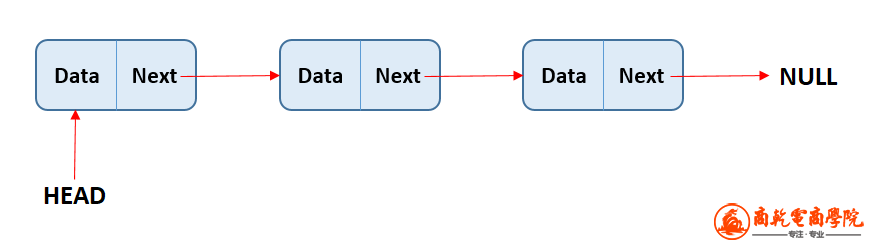6 t! Q+ q: ~# ]1 s( X
在单链表中每一个节点有单指针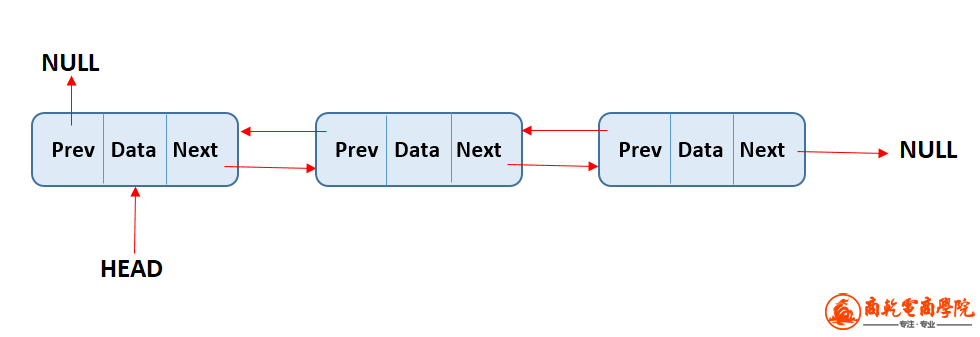在双链表中每一个节点有双指针列表的第一个元素被当作头，列表的最后一个元素被当作尾。和数组一样，列表的长度由列表中的元素个数决定。列表和数组主要不同包括：列表没有索引，列表中的每一个值仅“知道”其通过指针连接到的值。因为列表没有索引，所以我们不能随机访问列表中的元素。当我们想要访问一个值，必须通过从头到尾遍历整个列表的方法。没有索引的好处是添加或删除列表中任意部分比在数组中更高效。我们只需要重新分配指针指向的“相邻”值，但是在数组中，我们需要重新分配余下所有值的索引。和其他所有数据结构一样，可以采用不同的方法来操作以链表存储的数据。通常会使用：push（在尾部添加）、pop（在尾部删除）、unshift（在头部添加）、shift（在头部删除）、get（获取）、set（设置）、remove（删除）和 reverse（反转）。我们先来看看如何实现单链表，再来看看如何实现双链表。单链表完全实现单链表的代码如下：// 为列表中的每一个节点创建一个类1 }/ {" \6 D* O0 ?. X) k7 z3 i
class Node{
// 每一个节点有两个属性：其值和指向下一个值的指针
constructor(val){* ]- G. G( z( ]7 V. I! {  D# Q: ~
this.val = val
this.next = null
}/ f* V2 T8 E- k8 v' w3 D
}! z& K9 I\$ m. v% Z' f
8 U8 W" M# ~: @  d2 ~% K6 P5 j4 ~
//为列表创建一个类2 @" K; _6 u( }# a6 @9 d
class SinglyLinkedList{4 D- i  E. e9 G/ d8 Z9 D4 e
// 列表有三个属性，头、尾和列表大小
constructor(){! F' [5 @" ?) U6 z. Y
this.tail = null
this.length = 04 ^0 L: `9 Q5 N( a
}) K' X5 h6 W, y
// 向 push 方法传入一个值作为参数，并将其赋值给队列的尾
push(val) {- H* c& r8 N2 D# ~) B6 E
const newNode = new Node(val)
if (!this.head){1 k# K% b" M8 _' M% A* [; J
this.head = newNode. `# z4 r" n7 O& F; P8 [  c6 i
this.tail = this.head3 ~& `# {- ]5 x' V2 `" j
} else {. {4 ^\$ l2 F) K3 X2 A% I
this.tail.next = newNode
this.tail = newNode. t. H8 e  W+ n
}
this.length++
return this
}
// pop 方法删除队列尾: l# Y' f1 C+ J# W& A  k) Q
pop() {7 G# C# Z# k! U, u8 b8 G& \
if (!this.head) return undefined- a* Z9 Z9 ~2 a/ z
const newTail = current, z( f6 ^( v/ `  U" v' C. E! u
while (current.next) {
newTail = current, i+ K& \) S3 l/ X/ {
current = current.next
}  t6 A. I9 m" w, k
this.tail = newTail
this.tail.next = null, Q  n  M& r  C% B& @) j2 F, o
this.length--
if (this.length === 0) {0 H) q' B8 V\$ b, r+ s& }
this.tail = null
}
return current
}( k' ^- ]% a9 q2 G
// shift 方法删除队列头
shift() {* n) L\$ C! @% G: H1 i" ]\$ y% F0 z: r
this.length--
if (this.length === 0) {" n# s\$ {: L: Q! Z* \
this.tail = null
}  a3 z& [) O7 Z* o' O
}% ]& a' ~6 E: ^6 x( x- e2 f8 i
// unshift 方法将一个值作为参数并赋值给队列的头  y3 K& x( E: K9 h& L
unshift(val) {
const newNode = new Node(val)
this.head = newNode\$ \0 @! D* L& p% Q+ h- n; _1 K
}
newNode.next = this.head3 e. t\$ x! p  O
this.head = newNode/ u  `/ Z- H) J' h9 L/ r3 T
this.length++, I; w9 e' E8 `\$ v( K) j1 |+ H9 y, n/ q
return this
}
// get 方法将一个索引作为参数，并返回此索引所在节点的值
get(index) {
if(index < 0 || index >= this.length) return null8 v: s* ?+ H& t' h\$ ?
const counter = 0
while(counter !== index) {
current = current.next
counter++" \6 c! ]1 Z( }0 O
}
return current- O& R7 t5 d, v\$ E7 H. K9 [\$ I  j, F  b
}
// set 方法将索引和值作为参数，修改队列中索引所在的节点值为传入的参数值
set(index, val) {- \& `  R* ~' ]! K( w: ~( m  }
const foundNode = this.get(index)
if (foundNode) {
foundNode.val = val, H+ h! f, Y0 z- s8 j2 \" r/ S
return true
}8 u" u1 y\$ \; b2 N. {: Y
return false
}* R7 V- ]5 J' q3 a' k, I2 j* [
// insert 方法将索引和值作为参数，在队列索引位置插入传入的值& Q# I" N) J\$ _) F
insert(index, val) {
if (index < 0 || index > this.length) return false4 x: h5 l" H3 ?5 D, `9 f0 u
if (index === this.length) return !!this.push(val)) r4 b7 R, o9 s  P1 X' L) i3 E( P
if (index === 0) return !!this.unshift(val)

const newNode = new Node(val)
const prev = this.get(index - 1)\$ M! ~+ F5 w2 V
const temp = prev.next
prev.next = newNode
newNode.next = temp( g% \, c8 C4 m# W\$ Q) d: ~" {) P2 a
this.length++
return true
}
// remove 方法将索引作为参数，在队列中删除索引所在的值" a' p, s\$ K2 {; h( r
remove(index) {7 w" B3 T: q; a- a: ?: j2 C
if(index < 0 || index >= this.length) return undefined
if(index === 0) return this.shift()
if(index === this.length - 1) return this.pop()
const previousNode = this.get(index - 1)1 U6 {2 V+ o5 F% ~1 d! p
const removed = previousNode.next8 k3 Z( N, g# ~\$ E
previousNode.next = removed.next
this.length--  ]; ]9 K+ N; P
return removed' A* b3 C  j+ \& S\$ a
}1 y: K8 H2 p0 X. }
// reverse 方法反转队列和所有指针，让队列的头尾对调
reverse(){! }0 [3 V' o: D, F/ S
this.tail = node
let next. D- [# y: p5 p- S, w
const prev = null
for(let i = 0; i < this.length; i++) {
next = node.next. Y+ E& @& w) [& F
node.next = prev: z" b/ I! L  a5 P4 M
prev = node
node = next
}
return this3 Q! l! ?& N* T2 f" z2 r; m: }
}
}

class Node{" D% A* v/ l1 R, [9 m* p7 U5 q
// 每一个节点包含三个属性，其值，一个指向上一个节点的指针，一个指向下一个节点的指针
constructor(val){" I% |, i2 l! x) x' ]% _9 _3 u7 h
this.val = val;
this.next = null;
this.prev = null;
}
}

// 创建一个列表的类2 }, W; N. q/ d3 [/ S) ?
class DoublyLinkedList {1 K8 m8 @7 x, l* B8 f
// 列表有三个属性，头，尾和列表的大小, Y  X, e5 J1 H) W) Z3 ^/ P
constructor(){& A: L5 N% f2 s  y* Z* z
this.head = null/ U6 F. a) `8 u
this.tail = null+ ]6 V' i* ^8 n' H
this.length = 0\$ z% o9 L9 ?, Y9 \1 O" ^
}
// push 方法将值作为参数并赋值给队列尾1 X8 `3 K\$ B* x
push(val){( ^. @7 c/ V+ I  {8 C
const newNode = new Node(val)+ c, R  ]" E* s* a8 I  X* f
if(this.length === 0){
this.tail = newNode5 r8 O0 Q- n9 H3 m" r
} else {0 ~1 l\$ A) _3 J( W( M* M2 I' n; D\$ B
this.tail.next = newNode3 [% \  W! D# O  _. l6 b& }
newNode.prev = this.tail2 F" p, F% {4 X' B0 {
this.tail = newNode
}
this.length++
return this; {2 \0 n( ?7 }) v3 K: m
}
// pop 方法删除队列尾/ s) v' ?. _! y\$ @
pop(){1 F" c5 O  k: G  k- o& `) a; w
if(!this.head) return undefined% T, C7 p! B- T- [( @
const poppedNode = this.tail
if(this.length === 1){
this.head = null: a+ N; b* w1 A5 g& z& Z
this.tail = null
} else {6 C6 t\$ U+ m- R3 m; ?" \. [! M
this.tail = poppedNode.prev
this.tail.next = null/ I  |" |) ]  n+ ?. C
poppedNode.prev = null
}
this.length--
return poppedNode
}
// shift 方法删除队列头. i9 q, Z  @6 G
shift(){
if(this.length === 0) return undefined
if(this.length === 1){/ p9 y# c- X+ g1 f
this.tail = null. k6 s; d. M  I+ Y7 t
} else{0 n5 S' I; a8 i* I6 b( o! z0 T( i
oldHead.next = null( U; g: L3 T: s1 r0 F
}9 k5 w+ b5 {. m' c- H
this.length--" h) n3 U, Z/ o# M: M
return oldHead3 ^# X8 `% J( w- g4 B4 i; D
}
// unshift 方法将值作为参数并赋值给队列头! [- D& h6 `/ s: |4 M- E
unshift(val){5 q. m5 @7 g( k9 L! z9 \* A
const newNode = new Node(val)" q- N; F/ M+ E, S7 P% F7 p
if(this.length === 0) {
this.head = newNode: r+ c( ^# N- Z+ Q4 s  ?
this.tail = newNode& ], B# A9 o' j) C
} else {8 z" X/ S\$ b2 X1 \
this.head.prev = newNode8 s3 A: Y; e/ E% E
newNode.next = this.head7 o0 E5 e- _1 d- e
}2 \4 b& V/ T\$ v\$ O, N, N
this.length++
return this" Q4 i# q# `* F# @
}% e) s9 d* x5 U) P  u) X- F
// get 方法将索引作为参数并返回队列对应索引的值; n" `# a& N/ D, q7 r8 M5 v- o
get(index){: f/ J! _! R! D
if(index < 0 || index >= this.length) return null
let count, current. t. H! o  a4 j7 o( Y/ B" F; D# @; E
if(index <= this.length/2){
count = 0. t! T* o9 [9 {6 V# o/ ?\$ E& l
current = this.head, Y# c. N6 B; {/ u, \: v
while(count !== index){
current = current.next  l/ S. w' R' [8 z
count++. r; J. p" y; y; b
}
} else {
count = this.length - 1- ^4 O6 B& o  y9 X9 X. s& I
current = this.tail
while(count !== index){  {: n' u! ?+ w8 z5 \9 L6 {; r
current = current.prev
count--
}
}4 j2 q  F\$ H, O: G
return current
}
// set 方法将索引和值作为参数，修改队列中索引所在的节点值为传入的参数值+ {, H0 s. w% }, a4 U" G
set(index, val){
var foundNode = this.get(index)
if(foundNode != null){
foundNode.val = val
return true- p7 `0 ], S7 n. o; q. I
}
return false6 U9 i2 g7 k7 W/ Q
}
// insert 方法将索引和值作为参数，将值插入队列响应索引位置
insert(index, val){1 _& a1 ~6 d3 t4 X# W  ]
if(index < 0 || index > this.length) return false
if(index === 0) return !!this.unshift(val)8 y* \% H\$ L  K
if(index === this.length) return !!this.push(val)
" q& j* ^& g% A. o0 s6 M7 d
var newNode = new Node(val)
var beforeNode = this.get(index-1)4 K' j/ V8 |/ K! f9 G
var afterNode = beforeNode.next

beforeNode.next = newNode, newNode.prev = beforeNode7 `9 ?( ~6 w7 N
newNode.next = afterNode, afterNode.prev = newNode
this.length++
return true3 w* T8 n\$ m9 B( M: y\$ P
}
}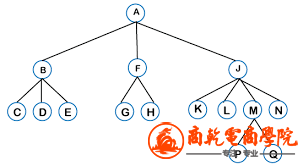树结构树由根节点（树的第一个节点）开始，其他所有由根发展出来的节点被称作子节点。树结构最底部的节点没有“后代”，被称为叶节点。树的高度由父子节点相连的层数决定。和链表及数组不同的地方是，树是非线性的，程序可以在数据结构内选择不同的方向遍历数据，从而得出不同的值。而在链表或者数组中，程序由一个端点开始遍历到另一端点，每一次都重复同样的路径。构成树结构一个重要的要素是仅从父到子连接的节点是合法的。“亲属”之间或者由子向父节点是我连接都不被允许（这样的连接会形成图表，是另一种数据结构），另一个重要的要素是树只能有一个根节点。程序中使用树的场景有：DOM 模型人工智能中的情景分析操作系统中的文件夹有不同类型的树，每一种类型的树的值都遵从不同的模式而组织起来，这样也就适用于不同的解决问题的场景。最常见的两种树是二叉树和堆。二叉树二叉树是每个节点最多只有两个节点的树结构。8 k4 g3 R% @* ^8 v" v\$ O" M6 l; H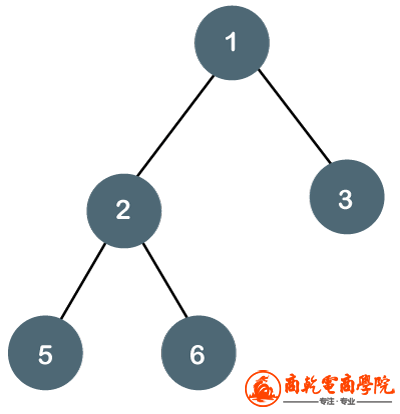二叉树二叉树的一个重要使用场景是搜索。用于搜索的二叉树被称为二叉查找树（BST）。BST 和普通二叉树类似，只是内部的数据结构被排列成易于搜索的结构。在 BST 中的值是排过序的，所有节点的左子节点的值要小于父节点，所有节点的右子节点的值要大于父节点。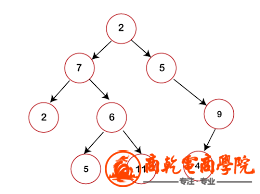二叉查找树这样给值排过序的数据结构非常适合做搜索，因为树的每一层都可以对比是比父节点大还是小，在对比的过程中，我们可以逐步舍弃掉一半的数据得到最终我们需要的值。当插入或者删除值的时候，我们的算法会进行如下步骤：检查是否存在根节点如果存在根节点，检查这个需要添加或删除的值是比根节点大还是小如果比根节点小，则检查左边是否有节点，并重复上面的步骤；如果左边没有节点，则将这个节点在当下位置添加或者删除如果比根节点大，则检查右边有没有节点，并重复上述步骤；如果有变没有节点，则将这个节点在当下位置添加或者删除在 BST 中查找与上述方法类似，但是没有添加或者删除值，取而代之的是与节点比较我们搜寻的值的大小。树的大 O 复杂度呈对数（log（n））。但是需要注意的是，想要实现这样的时间复杂度，必须保证树结构的每一步都是左右对称的，这样我们才可以在搜索的过程中“丢弃”一半的数据。如果在任意一边存储的值更多，树结构的搜索效率就会打折扣。实现 BST 的方法如下：// 我们创建树的节点
class Node{
// 每一个节点有三个属性：其值，以及指向左节点的指针和指向右节点的指针4 w7 h" ?' y% z* x* L8 e* u
constructor(value){# ]  u+ p5 P7 R\$ B% h, x
this.value = value
this.left = null
this.right = null
}: m) w3 s1 u7 F! F9 U
}1 V8 ^' O1 H1 n4 v
// 创建BST的类1 W# u9 P8 X" C7 Z+ {
class BinarySearchTree {5 a- b+ {" I\$ d7 _4 o, y  ~
// 这个树只有一个属性即根节点
constructor(){
this.root = null: c7 W( o9 M5 _  v. q
}
// insert 方法将一个值作为参数，并将值插入树对应的位置
insert(value){
const newNode = new Node(value)
if(this.root === null){
this.root = newNode! d0 i; q* e3 `
return this
}5 L6 I2 \/ X  n) t  M
let current = this.root
while(true){
if(value === current.value) return undefined
if(value < current.value){
if(current.left === null){
current.left = newNode( c; l* ~( A- ~. v1 A4 }
return this" ?) b3 q/ V; y+ m" M/ O/ E
}
current = current.left
} else {
if(current.right === null){
current.right = newNode
return this- Z3 P9 @! R- o) K" \2 q' `, j
}
current = current.right
}0 P/ w9 o7 `  {* B/ s
}
}
// find 方法将值作为参数，遍历树寻找对应的值8 ^. h5 n5 F+ R8 }
// 如果找到了，返回找到的值，如果没有找到，返回undefined4 N, h+ K" r- P6 }
find(value){
if(this.root === null) return false* z) I6 L4 }6 W7 b3 d8 E
let current = this.root,
found = false! }( ?7 X8 O* n: j
while(current && !found){
if(value < current.value){
current = current.left
} else if(value > current.value){
current = current.right; S1 c, F  y  M' j
} else {
found = true/ j. v8 i( o  X. f6 [9 s; x% _# A, E
}/ a5 ?8 M' H- Z. C- Z
}- T( X/ ?\$ r& `  C5 @7 v: i* U& b
if(!found) return undefined% z/ ^2 A\$ h! b5 ]
return current8 J\$ j; C6 H# i7 t" d
}4 K5 K7 {3 b1 K& Q1 [! W
// contain 方法将值作为参数，如果找到树中对应的值返回 true，如果没有找到则返回 false
contains(value){" t2 _9 a% y& \* V! _
if(this.root === null) return false+ {% k: ~' ^: @\$ F& l- {
let current = this.root,8 C2 b. E: P! c& Z1 a
found = false; _8 r& E5 O2 p2 ]# g! N
while(current && !found){\$ I% j. y1 K6 a- X# R' i
if(value < current.value){1 E) W+ l( j: ^; r: W- o
current = current.left
} else if(value > current.value){; |. _: Y2 c& C1 \4 S" |  {
current = current.right  s/ Y* `+ W2 q: \
} else {
return true
}+ p. P, Q" s8 F' J; [7 l
}
return false1 q" u' ^& T0 z/ H
}
}8 P9 v& l9 x8 U# l\$ f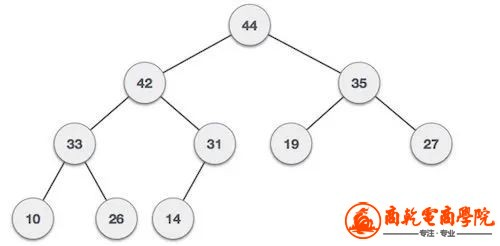最大堆" a  L% t+ A  H( W. @' [+ a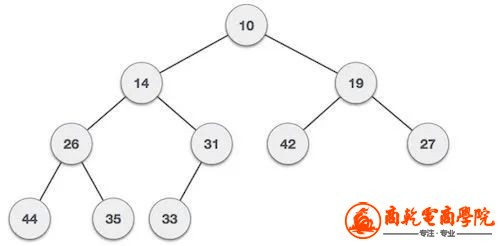最小堆堆结构的规则不适用于相邻的两个节点，也就是说在同一层的节点除了必须比自己的父节点大或者小，不需要遵循其他规则。另外，堆越紧凑越好，也就是每一层都尽可能填满空位，新的节点首先添加到左边。堆，特别是二进制堆，通常被用来解决优先队列问题，也被运用到知名的算法问题——戴克斯特拉算法。优先队列是一种数据结构，在这种结构中，每一个元素都被关联了优先级，优先级高的元素优先展示出来。图图是一种有一组节点相互连接的数据结构。和树不一样的是，图并没有根或者叶节点，也没有“头”或者“尾”。不同的节点随机关联在一起，之间并没有父子关系。3 F6 ~, P+ _) r! D( @& g& M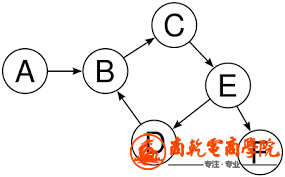\$ G4 }# b. g# r
图图经常被应用于：社交网络地理定位推荐系统根据节点之间关联的特征，可以把图分成不同的类别：有向图和无向图如果节点之间没有的关联没有定义方向，我们就称这个图为无向图。在下图中我们可以看到节点 2 和节点 3 之间的关联没有方向性，我们可以从节点 2 到节点 3，也可以从节点 3 到节点 2。无定向意味着节点间的连接是双向的。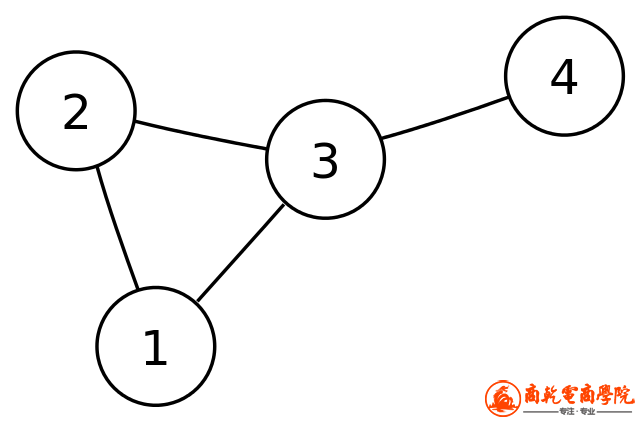! u6 k- K9 F! y0 p+ H
无向图你可能已经猜出来了，有向图就是完全相反的。让我们再次使用上面的图，这时节点之间的连接是有固定方向的。在这幅图中，你可以由节点 A 到节点 B，但是不能从节点 B 到节点 A。

有向图加权图和非加权图如果节点之间的连接被分配了权重，我们就称其为加权图。权重仅分配给了节点之间的连接，仅和连接相关，不和节点相关。在下面的例子中我们可以看到，节点 0 和节点 4 之间连接的权重是 7；而节点 3 和节点 1 之间的权重是 4。8 P  r& K; z  u7 i  P! g+ M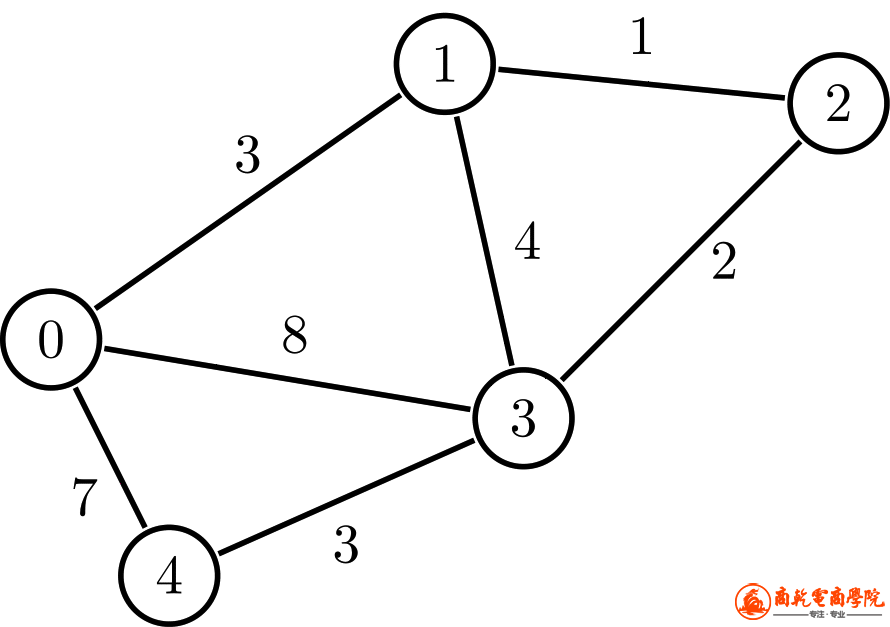加权图想要了解加权图，可以想象你需要向用户展现一个标注了不同地点的地图，你需要告诉用户从一个地方到另一个地方需要花多长时间。加权图就可以用来表现这个场景，你可以使用节点来存储地点的信息，节点之间的连接就是两个地点之间的道路，连接的权重代表从一个地点到另一个地点的物理距离。% B1 C1 W  u, I" u4 O9 O! R4 D* ~' I
加权图被大量应用在定位系统你应该已经猜到了，无加权图即节点之间的连接没有被分配权重，所以节点间的连接没有额外的信息，只表达节点间的关系。如何表达图在编码图的时候，主要可以使用两种方法：邻接矩阵和邻接列表。让我们分别看看这两种方法的优缺点。邻接矩阵是一个二维结构代表图的节点和节点之间的连接。如果我们使用这个的例子：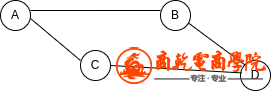! f6 _% q9 M, \) C( f+ I5 W3 x% ]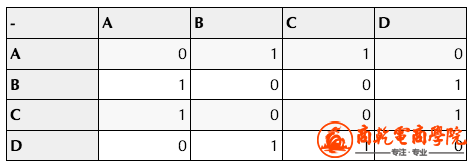[0, 1, 1, 0]
[1, 0, 0, 1]
[1, 0, 0, 1]
[0, 1, 1, 0]
]6 R4 ~1 k& b2 z& u\$ @9 ]6 Y# k

A: ["B", "C"],
B: ["A", "D"],3 r  K. G; G: K& m
C: ["A", "D"],. F/ b0 g: s* `8 Z, M2 T" q, O- m
D: ["B", "C"],
}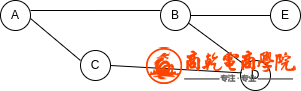/ }/ p0 N. R( _( `# s' ]0 U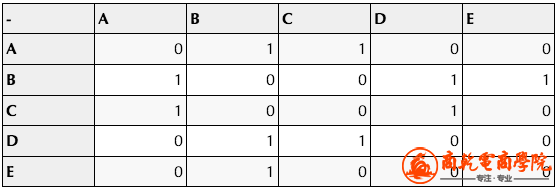{
A: ["B", "C"],% c4 S, w% J/ y" \0 v7 g\$ K
B: ["A", "D", "E"],& r4 p\$ v7 U8 n6 M
C: ["A", "D"],
D: ["B", "C"],2 R7 p7 {! |5 m) _. r
E: ["B"],. B\$ f  J5 {\$ v: x1 f% s\$ I
}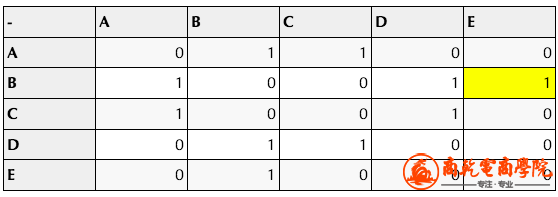& w. |\$ G1 a. F9 e

class Graph{
// 图仅有一个属性，即邻接列表
constructor() {
this.adjacencyList = {}4 G1 D9 \, J% s( h3 Y# u+ ^5 D
}
}4 ?) h1 ^# s2 ~! A
// addConnection 将两个节点作为参数，并添加到每一个节点键对应的值的数组中5 j& X4 p* L' A. J" V) U' [7 w
this.adjacencyList[node1].push(node2)+ v" @9 b; Y" D( i% k- W: N' k
}+ V2 }% P4 R3 i- q% f
// removeConnection 方法将两个节点作为参数，并删除掉非自己节点对应数组里的值
removeConnection(node1,node2) {
this.adjacencyList[node2] = this.adjacencyList[node2].filter(v => v !== node1)+ r4 e  m9 ~7 a, O2 q
}
// removeNode 方法将节点作为参数，删除该节点所有的连接，并且删除列表中该节点相关的键8 m& k% P\$ c, N* G9 g! g2 X* P: b% p
removeNode(node){/ K6 ?2 |. q0 x* b
this.removeConnection(node, adjacentNode)2 {! k3 K8 J) H# T/ ]
}2 M* O% I% }2 r8 a( M4 Y: r
delete this.adjacencyList[node]! ^- J0 v: D3 ]6 K\$ L
}6 \* O3 c/ |7 R" E# J% }
}
+ {\$ u" G* y+ V# O: \  B
const Argentina = new Graph()6 k7 b9 ?2 m- Y. s' Q& ~' _
Argentina.addNode("Buenos Aires")  ~' k5 G' M/ q2 _) B& ?
Argentina.addNode("Santa fe")% ~4 B7 Z: N  O
Argentina.addNode("Mendoza")  e6 r# c8 A0 G: M# K* u3 q/ J( ]+ C

console.log(Argentina)
// Graph {
//     adjacencyList: {0 Y; H/ r% H! L, D. l6 d
//         'Buenos Aires': [ 'Córdoba', 'Mendoza' ],  L4 X& t# {) z% d. N
//         'Santa fe': [ 'Córdoba' ],
//         'Córdoba': [ 'Buenos Aires', 'Santa fe' ],
//         Mendoza: [ 'Buenos Aires' ]
//     }
// }) p; B" ?) o1 A& h" z### 相关帖子本版积分规则 回帖并转播 回帖后跳转到最后一页•官方手机版

•微信公众号

•商务合作